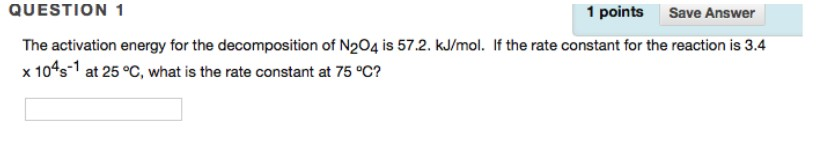# Problem: Question 1The activation energy for the decomposition of N2O4 is 57.2. kJ/mol. If the rate constant for the reaction is 3.4 x 104s-1 at 25 °C, what is the rate constant at 75 °C?

###### FREE Expert Solution
87% (256 ratings)###### Problem Details

Question 1

The activation energy for the decomposition of N2O4 is 57.2. kJ/mol. If the rate constant for the reaction is 3.4 x 104s-1 at 25 °C, what is the rate constant at 75 °C?